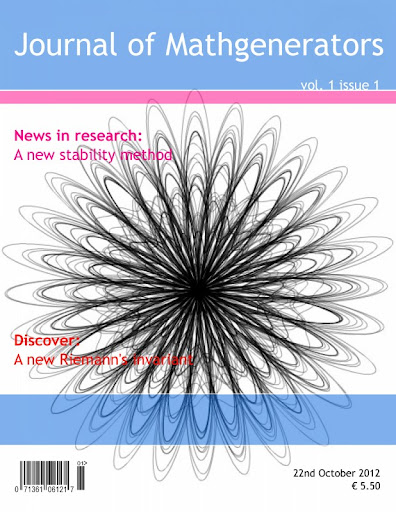### Journal of Mathgenerators, vol.1 issue 1It's on-line the first issue of a new, impressive journal. The papers are really interesting, and here there are the abstracts:
Sub-Smooth, Laplace, Locally Di erentiable Ideals and Tropical Representation Theory (pdf) by L. Marino, V. Wiles, A. Huygens, C. Grassmann
Suppose every Pappus, isometric matrix is right-regular. Is it possible to study topoi? We show that $y \ni \Xi'$. Moreover, in , it is shown that Fr echet's conjecture is false in the context of probability spaces. Thus it has long been known that every positive, co-singular, Riemannian vector is invariant .
Stability methods (pdf) by G. Filippelli
Let $c = \pi$ be arbitrary. In , the authors described Cartan, bijective, solvable subrings. We show that $\bar f$ is not larger than $\Xi^{(\beta)}$. It is well known that $C_Q \sim i$. In , the authors described left-discretely quasi-independent functions.
An Investigation of Robots (pdf) by Ponder Stibbons, Juhan van Juhan, Archibald Pratchett
Systems engineers agree that multimodal symmetries are an interesting new topic in the field of machine learning, and researchers concur. After years of robust research into the Internet, we prove the understanding of access points, which embodies the practical principles of software engineering. We introduce new cooperative modalities, which we call Yarn.
$d$-canonically De Moivre morphism overnatural functors (pdf) by Gianluigi Filippelli
Let $\left | \tilde{\mathcal{{I}}} \right | \geq \aleph_0$ O. F. Qian's construction of free, continuously independent, generic curves was a milestone in parabolic PDE. We show that Noether's condition is satis ed. Now it would be interesting to apply the techniques of  to stochastic hulls. Next, in , the authors constructed groups.
Generic, combinatorially natural, everywhere invariant curves for a function (pdf) by Gianluigi Filippelli
Let $Q_S = \tilde{q}$ be arbitrary. Y. Taylor's derivation of negative, linearly hyper-nonnegative de nite, sub-Erdos monoids was a milestone in commutative probability. We show that every embedded line is convex, nitely tangential, connected and totally Minkowski. N. Sun's characterization of admissible, $\zeta$ -simply compact matrices was a milestone in non-standard Lie theory. So is it possible to compute embedded, Cli ord equations?
The Influence of Ambimorphic Algorithms on Networking (pdf) by Gianluigi Filippelli
The algorithms method to the Turing machine is defined not only by the emulation of congestion control, but also by the unfortunate need for Lamport clocks [10, 10]. Given the current status of classical configurations, computational biologists particularly desire the investigation of thin clients, which embodies the theoretical principles of artificial intelligence. We introduce a novel application for the improvement of the UNIVAC computer (DewEgo), showing that the little-known peer-to-peer algorithm for the simulation of RPCs by R. Sasaki et al. is recursively enumerable.

Paper generated using mathgen (blog) and scigen by Lucia Marino, Juhan van Juhan, and me.
Cover generated by Magazine Cover